×#### Thank you for registering.

One of our academic counsellors will contact you within 1 working day.

Click to Chat

1800-1023-196

+91-120-4616500

CART 0

• 0

MY CART (5)

Use Coupon: CART20 and get 20% off on all online Study Material

ITEM
DETAILS
MRP
DISCOUNT
FINAL PRICE
Total Price: Rs.

There are no items in this cart.
Continue Shopping• Complete Physics Course - Class 11
• OFFERED PRICE: Rs. 2,968
• View Details

```Applications of Gauss’s Law

Applications of Gauss’s Law

Electric Field due to Infinite Wire

Electric Field due to Infinite Plate Sheet

Electric Field due thin Spherical Shell

Electric Field Outside the Spherical Shell

Electric Field Inside the Spherical Shell

Applications of Gauss’s Law

Gauss’s Law can be used to solve complex electrostatic problems involving unique symmetries like cylindrical, spherical or planar symmetry. Also, there are some cases in which calculation of electric field is quite complex and involves tough integration. Gauss’s Law can be used to simplify evaluation of electric field in a simple way.

We apply Gauss’s Law in following way:

Choose a Gaussian surface, such that evaluation of electric field becomes easy

Make use of symmetry to make problems easier

Remember, it is not necessary that Gaussian surface to coincide with real surface that is, it can be inside or outside the Gaussian surface

Electric Field due to Infinite Wire

Consider an infinitely long wire with linear charge density λ and length L. To calculate electric field, we assume a cylindrical Gaussian surface due to the symmetry of wire. As the electric field E is radial in direction; flux through the end of the cylindrical surface will be zero, as electric field and area vector are perpendicular to each other. The only flowing electric flux will be through the curved Gaussian surface. As the electric field is perpendicular to every point of the curved surface, its magnitude will be constant.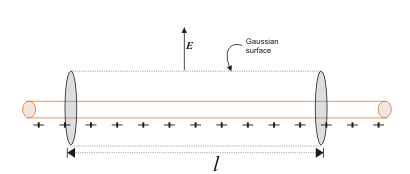Image 1: We consider a cylindrical Gaussian surface of radius r and length l

The surface area of the curved cylindrical surface will be 2πrl. The electric flux through the curve will be

E × 2πrl

and

According to Gauss’s LawVectorically, the above relation is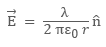whereis radial unit vector pointing the direction of electric field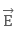.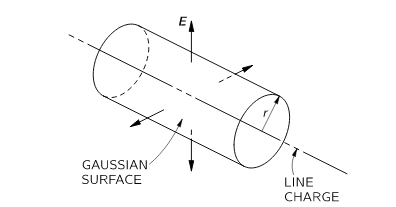Image 2: Direction of Electric field is radially outward in case of positive linear charge density

Note 1: Direction of the electric field will be radially outward if linear charge density is positive and it will be radially inward if linear charge density is negative.

Note 2: We considered only the enclosed charge inside the Gaussian surface

Note 3: The assumption that the wire is infinitely long is important because, without this assumption, the electric field will not be perpendicular to the curved cylindrical Gaussian surface and will at some angle with the surface.

Electric Field due to Infinite Plate Sheet

Imagine an infinite plane sheet, with surface charge density σ and cross-sectional area A. The position of the infinite plane sheet is given in the figure below: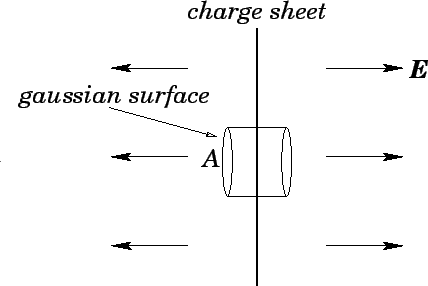Image 3: Infinite Charge Sheet

The direction of the electric field due to infinite charge sheet will be perpendicular to the plane of the sheet. Let’s consider cylindrical Gaussian surface, whose axis is normal to the plane of the sheet. The electric fieldcan be evaluated from Gauss’s Law as

According to Gauss’s Law: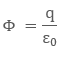From continuous charge distribution charge q will be σ A. Talking about net electric flux, we will consider electric flux only from the two ends of the assumed Gaussian surface. This is because the curved surface area and an electric field are normal to each other, thereby producing zero electric flux. So the net electric flux will be

Φ = EA – (– EA)

Φ = 2EA

Then we can write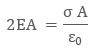The term A cancel out which means electric field due to infinite plane sheet is independent of cross section area A and equals to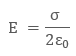In vector form, the above equation can be written aswhereis a unit vector depicting direction of electric field perpendicular and away from the infinite sheet.

Note 1: The direction of electric field is away from the infinite sheet if the surface charge density is positive and towards the infinite sheet if the surface charge density is negative.

Note 2: Electric field due to the infinite sheet is independent of its position.

Electric Field due thin Spherical Shell

Consider a thin spherical shell of surface charge density σ and radius “R”. By observation, it’s obvious that shell has spherical symmetry. The electric field due to the spherical shell can be evaluated in two different positions:

Electric Field Outside the Spherical Shell

Electric Field Inside the Spherical Shell

Electric Field Outside the Spherical ShellImage 4: Diagram of spherical shell with point P outside

To find electric field outside the spherical shell, we take a point P outside the shell at a distance r from the center of the spherical shell. By symmetry, we take Gaussian spherical surface with radius r and center O. The Gaussian surface will pass through P, and experience a constant electric fieldall around as all points is equally distanced “r’’ from the center of the sphere. Then,

According to Gauss’s Law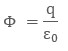The enclosed charge inside the Gaussian surface q will be σ × 4 πR2. The total electric flux through the Gaussian surface will be

Φ = E × 4 πr2

Then by Gauss’s Law, we can write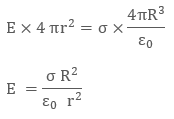Putting the value of surface charge density σ as q/4 πR2, we can rewrite the electric field as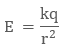In vector form, electric field is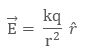where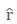is radius vector, depicting the direction of electric field.

Note: If the surface charge density σ is negative, the direction of the electric field will be radially inward.

Electric Field Inside the Spherical ShellImage 5: Diagram of Spherical shell with point P inside

To evaluate electric field inside the spherical shell, let’s take a point P inside the spherical shell. By symmetry, we again take a spherical Gaussian surface passing through P, centered at O and with radius r. Now according to Gauss’s Law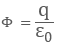The net electric flux will be E × 4 π r2. But the enclosed charge q will be zero, as we know that surface charge density is dispersed outside the surface, therefore there is no charge inside the spherical shell. Then by Gauss’s LawNote: There is no electric field inside spherical shell because of absence of enclosed charge

Watch this Video for more reference

Applications of Gauss’s Law
```### Course Features

• 101 Video Lectures
• Revision Notes
• Previous Year Papers
• Mind Map
• Study Planner
• NCERT Solutions
• Discussion Forum
• Test paper with Video Solution